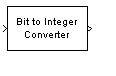# Bit to Integer Converter

Map vector of bits to corresponding vector of integers

•Libraries:
Simulink / Logic and Bit Operations
Communications Toolbox / Utility Blocks

## Description

The Bit to Integer Converter block maps groups of bits in the input vector to integers in the output vector.

If M is specified by the Number of bits per integer(M) parameter:

• For unsigned integers, the block maps each group of M bits to an integer in the range [0, (2M – 1)]. As a result, the output vector length is 1/M times the input vector length.

• For signed integers, the block maps each group of M bits to an integer in the range [(–2M-1), (2M – 1 – 1)].

## Ports

### Input

expand all

Input signal, specified as a scalar or column vector of bits with a length that is a multiple of the value specified in the Number of bits per integer(M) parameter. The input must be bits with values of 0 or 1.

Data Types: `double`

### Output

expand all

Output signal, returned as an integer or column vector of integers. The After bit packing, treat resulting integer values as parameter specifies whether input bits are treated as unsigned or signed.

• When the input bits are treated as unsigned, each integer output is in the range [0, (2M – 1)].

• When the input bits are treated as signed, each integer output is in the range [(–2M-1), (2M – 1 – 1)].

## Parameters

expand all

Number of input bits mapped to each integer in the output, specified as an integer in the range [1, 32].

#### Programmatic Use

 Block Parameter: `nbits` Type: character vector Values: integer in the range [1, 32] Default: `'3'`

Input bit order, specified as `'MSB first'` or `'LSB first'`.

• `'MSB first'` –– First bit of the input signal is the most significant bit (MSB).

• `'LSB first'` –– First bit of the input signal is the least significant bit (LSB).

#### Programmatic Use

 Block Parameter: `bitOrder` Type: character vector Values: ```'MSB first'``` | `'LSB first'` Default: ```'MSB first'```

Specify whether the resulting integer values are treated as signed or unsigned after bit packing. This parameter setting determines which Output data type selections are available.

Tip

When this parameter is set to `Unsigned` and the block has an overflow, the block behaves as though After bit packing, treat resulting integer values as is set to `Signed`.

#### Programmatic Use

 Block Parameter: `signedOutputValues` Type: character vector Values: `'Unsigned'` | `'Signed'` Default: `'Unsigned'`

The Output data type options change depending on the desired signedness of the output.

If the output integers are `Signed`, you can choose from the following Output data type options:

• `Inherit via internal rule`

• `Smallest integer`

• `double`

• `single`

• `int8`

• `int16`

• `int32`

If the output integers are `Unsigned`, you can choose from the following options in addition to the `Signed` options:

• `Same as input`

• `uint8`

• `uint16`

• `uint32`

When you set the parameter to ```Inherit via internal rule```, the block determines the output data type based on the input data type.

• If the input signal is floating-point (either `double` or `single`), the output data type is the same as the input data type.

• If the input data type is not floating-point, the output data type is determined as if the parameter is set to `Smallest integer`.

When you set the parameter to ```Smallest integer```, the block selects the output data type based on the settings used in the Hardware Implementation Pane of the Configuration Parameters dialog box.

• If you select `ASIC/FPGA` for the device vendor, the output data type is the smallest ideal integer or fixed-point data type, based on the setting for the Number of bits per integer(M) parameter.

• For all other device vendor selections, the output data type is the smallest available (signed or unsigned) integer word length that is large enough to fit the ideal minimum bit size.

#### Programmatic Use

 Block Parameter: `outDtype` Type: character vector Values: ```'Inherit via internal rule'``` | `'Smallest integer'` | `'Same as input'` | `'double'` | `'single'` | `'int8'` | `'uint8'` | `'int16'` | `'uint16'` | `'int32'` | `'uint32'` Default: ```'Inherit via internal rule'```

## Block Characteristics

 Data Types `Boolean` | `double` | `fixed pointa, b` | `integer` | `single` Direct Feedthrough `no` Multidimensional Signals `no` Variable-Size Signals `yes` Zero-Crossing Detection `no` a Fixed-point inputs must be ufix(1).b ufix(N) or sfix(N) when ASIC/FPGA is selected in the Hardware Implementation Pane and output data-type is set to either (a) Smallest integer or, (b) Inherit via internal rule and at the same time input is non floating-point.

## Version History

Introduced before R2006a

expand all